Next: About this document ... Up: COMP9417 Project Shapiro's Model Previous: Bibliography

# Logic and Logic Programming Definitions

Most of these definitions follow Lloyd [Llo87b].

Unconstrained resolution, while an improvement on its predecessors, is still a massively non-deterministic search through a very large space of possible proofs. Interestingly, there is a class of clauses - the definite clauses - over which a variant of resolution can be made quite efficient.

Assume or give a brief overview of most-general-unifiers. A good overview of resolution theorem proving and its relationship to logic programming is [Rob92].

Atom:
If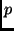is a predicate symbol and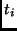are terms, then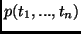is an atom. Note this is at odds with the builtin meta-predicates atom and atomic.

Chronological backtracking:
In Prolog, a failing computation causes a re-examination of the most-recent choice of the clause to execute, in a context where there were several alternatives. The computation proceeds with some other selection.

Clause:
A clause is a logical formula in conjunctive normal form, which has the schema: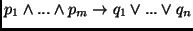or, equivalently,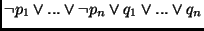where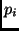andare atoms.

Clocksin and Mellish [CM81, Appendix B] give a Prolog program to convert arbitrary first-order formulae into clausal form.

Functor:
A function symbol/arity pair. These have the same role as records in other languages (structs in C) which is to aggregate data. These are the data constructors in the context of type systems.

Ground:
A term or atom is ground if it contains no variables.

Horn Clause:
A clause containing at most one positive literal, written (with the commas interpreted as):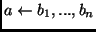(a definite clause) or(a definite goal). Note that introducing negated body goals into the language violates this definition.

Instance of:
One term is an instance of another if it is more instantiated than it, in the sense that it is the result of applying some substitution to the other.

Formally: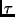is an instance of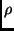if there exists a substitution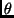such that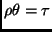, written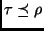.

Mode:
instantiation states - i.e. whether a variable is bound or free, or in terms of predicates, whether an argument position is an input or an output (or both). Note that the instantiation state of a term can be arbitrarily complex.

Module System:
Some mechanism for dividing a program into separate compilation units, for the purposes of abstraction.

Negation-as-finite-failure:
Prolog uses the closed-world-assumption: if it cannot prove a goal using the clauses in it's database, and the proof attempt fails after a finite number of steps, then the goal is deemed to be false. This rule means that Prolog implements a non-monotonic logic: it is not necessarily the case that adding more clauses to the theory will lead to more things being provable. This is in contrast to classical first-order logic, which is monotonic.

Predicate Symbol:
A predicate symbol is a string/arity pair that names a set of clauses (all of which have the same predicate symbol as heads).

Pure Prolog:
Restriction of the language to Horn clause logic - i.e. no higher-order constructs (call/1, findall/3, ...), meta-predicates (functor/3, arg/3, ...) or built in arithmetic (is/2, ...).

Resolution:
A sound and complete proof technique for first-order clausal logic, invented by Robinson in the early 1960's [Rob92]. Prolog uses a variant of full resolution, where constraints are imposed on clauses and the inputs to the resolution step, called SLD-NF resolution (linear resolution over definite clauses with a selection function, combined with the non-monotonic negation-as-failure rule [Llo87b]).

Substitution:
A substitution is a function that maps a set of variables to a set of terms, and is usually denoted. We writeto indicate that the termresults when we apply substitutionto term.

Term:
A term is one of: a variable, a constant symbol (e.g. a numeral), or a compound structurewhere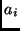are terms. In the latter case,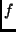is called a function symbol,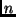is the arity of the term, and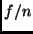is the functor.

Type:
A type is a collection of objects deemed to have some common property, such as the set of natural numbers.

Unification:
Unification can be viewed as a generalisation of pattern matching (such as that found in Haskell) in that variables can be bound in both the pattern and the object matched against, rather than just the pattern.

Unification is used in Prolog for assignment, memory allocation, pattern matching, argument binding, etc. As such it can be heavily optimised provided we can discover (essentially) mode information.

More formally, we assume the existence of a sound method of finding the most general unifier (mgu) of two terms, written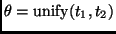. See [Llo87b] for more details.

Variants:
Two terms are variants if they are identical modulo a variable renaming, which is analogous to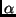-convertibility in the lambda calculus.

Ifandare variants, we write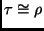. Obviously.Next: About this document ... Up: COMP9417 Project Shapiro's Model Previous: Bibliography
Peter Gammie
2002-03-01